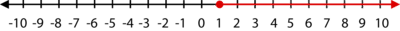# 3.4: Inequality Expressions

•• Contributed by CK12
• CK12

## Inequality Expressions

Suppose you're having a party, and you know that the number of people attending will be greater than or equal to 25. How would you write this inequality? If you had to graph the solutions to this inequality on a number line, could you do it? .

### Inequality Expressions

An algebraic inequality is a mathematical sentence connecting an expression to a value, a variable, or another expression with an inequality sign.

Verbs that translate into inequalities are:

> “greater than”

≥ “greater than or equal to”

< “less than”

≤ “less than or equal to”

≠ “not equal to”

Solutions to one-variable inequalities can be graphed on a number line or in a coordinate plane.

Inequalities that “include” the value are shown as ≤ or ≥. The line underneath the inequality stands for “or equal to.” We show this relationship by coloring in the circle above this value on the number line. For inequalities without the “or equal to,” the circle above the value on the number line remains unfilled.

#### Let's graph the solutions to t>3 on a number line:

The inequality is asking for all real numbers larger than 3.

#### Now, let's write the inequality pictured below:

The value of four is colored in, meaning that four is a solution to the inequality. The red arrow indicates values less than four. Therefore, the inequality is:

x≤4

#### Expressing Solutions to Inequalities

There are four ways to express solutions to inequalities:

1. Inequality notation: The answer is expressed as an algebraic inequality, such as d≤12.
2. Set notation: The inequality is rewritten using set notation brackets { }. For example, {d|d≤12} is read, “The set of all values of d, such that d is a real number less than or equal to one-half.”
3. Interval notation: This notation uses brackets to denote the range of values in an inequality.
1. Square or “closed” brackets [ ] indicate that the number is included in the solution
2. Round or “open” brackets ( ) indicate that the number is not included in the solution.

Interval notation also uses the concept of infinity ∞ and negative infinity −∞. For example, for all values of d that are less than or equal to 12, you could use set notation as follows: (−∞,12].

1. As a graphed sentence on a number line.

#### Let's describe the set of numbers contained by the given set notation for the following:

1. (8, 24)

(8, 24) states that the solution is all numbers between 8 and 24 but does not include the numbers 8 and 24.

1. [3, 12)

(3, 12) states that the solution is all numbers between 3 and 12, including 3 but not including 12.

### Examples

Example 3.4.1

Earlier, you were told to suppose that you're having a party where the number of people attending will be greater than or equal to 25. How would you write this inequality? Could you graph it?

Solution

Remember that the symbol that translates to "greater than or equal to " is ≥. Let p represent the number of people attending the party. Then, the inequality that represents this situation is:

p≥25

The graph of this inequality would have a solid dot at 25 and have an arrow moving to the right since the number of people attending is greater than or equal to 25.

Example 3.4.2

Describe and graph the solution set expressed by (∞,3.25).

Solution

The solution set contains all numbers less than 3.25, not including 3.25.

The graph on the number line is:

### Review

1. What are the four methods of writing the solution to an inequality?

Graph the solutions to the following inequalities using a number line.

1. x<−3
2. x≥6
3. x>0
4. x≤8
5. x<−35
6. x>−17
7. x≥20
8. x≤3

Write the inequality that is represented by each graph.

1.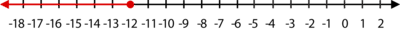2.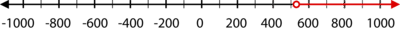3.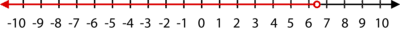4.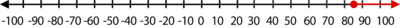5.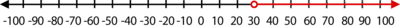6.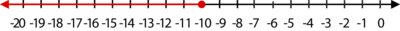7.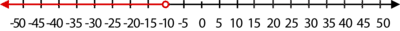8.